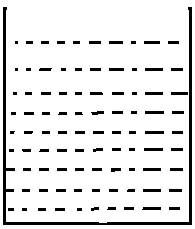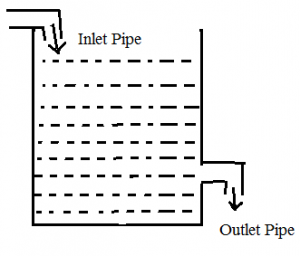# PIPES AND CISTERN PART 1-LEARN SERIES

Hi Bankersdaily Aspirants,

Learning is a step by step process and you should gradually progress in that to ace the process. As you all know we have started a new Learn Series to help with your preparations for various exams like IBPS PO , IBPS RRB Scale I officer , IBPS RRB Office Assistant , IBPS Clerk , IBPS SO , SBI PO , SBI Clerk , SBI SO and many other exams in this type. Nevertheless this series will also contribute you all the tips and tricks to every miscellaneous sections and also all the important topics which are asked in the exams. If you haven’t looked at the Learn series , Please Click the below link to learn the new concepts.

## INTRODUCTION TO PIPES AND CISTERN:

Today,we are going to look on Pipes and Cisterns.Before we are getting into the topic look into Time and Work because the problems based on Pipes and Cistern is also solved by using LCM Method which we used to solve  Time and Work.

## CISTERN:

Cistern is referred to a tank/Reservoir which has some specific capacity to hold liquid.## PIPES:There are two types of Pipes,They are

1)Inlet Pipe

2)Outlet Pipe

Inlet Pipe:

Inlet Pipe is used to fill the tank.

Outlet Pipe:

Outlet Pipe is used to empty the tank.

There may be any number of Inlet Pipes and Outlet Pipes that are connected to a cistern.

Before Getting into this topic,let us remember 3 basic terms

Total Capacity=LCM(Given Numbers)(unit-Litres)

Time=Total Capacity/Efficiency(unit-hr,sec,min,day)

Efficiency=Total Capacity/Time(unit Litre/hour)

## #.1.TYPE 1:

### PROBLEMS BASED ON INDIVIDUAL CAPACITY:

There are 3 sub type in this type,they are

1)When both the pipes are Inlet Pipes

2)When both the pipes are Outlet Pipes

3)One Pipe is Inlet Pipe and Outlet Pipe

1)When both the pipes are Inlet Pipes:

1)Pipe A can fill the tank in 10 hrs and Pipe B can fill the tank in 40 hrs.How long will it take to fill the tank if both the pipes are opened?

Explanation

Given that,

Pipe A can fill the tank in 10 hrs and Pipe B can fill the tank in 40 hrs

Total Capacity=LCM(10,40)=40Litres

A’s Efficiency=40/10=4 litre/hour

B’s Efficiency=40/40=1 litre/hour

So,

When both are opened,their total capacity=4+1=5 Litre/hour

Time=40/5=8 hours

So,When Pipe A and B are opened together,they can fill the tank in 8 hours

2)When both the pipes are Outlet Pipes:

1)Pipe A can empty the tank in 3 hours where as Pipe B can empty the tank in 6 hours.When they opened together how,long will it take to empty the tank?

Explanation

Given that,

Pipe A can empty the tank in 3 hours where as Pipe B can empty the tank in 6 hours.

Total Capacity of the tank=LCM(3,6)=6 Litres

A’s Efficiency=6/3=2litres/hour

B’s Efficiency=6/6=1 litre/hour

Total Efficiency of (A+B)=(2+1=3litres/hour)

Time=6/3=2hours

Therefore,When A & B are opened together,they can empty the tank in 2 hours

3)One Pipe is Inlet Pipe and Outlet Pipe:

1)A can fill the tank in 12 hours,B can empty the tank in 15 hours.When both the pipes are opened find how long will it take to empty/fill the tank ?

Explanation

Given that,

A can fill the tank in 12 hours,B can empty the tank in 15 hours

Total Capacity=LCM(12,15)=60 Litres

A’s(Inlet Pipe) Efficiency=60/12=5litre/hour

B’s(Outlet Pipe)Efficiency=60/15=4litre/hour

Note:

If the Efficiency of Inlet Pipe is greater then the Outlet Pipe the tank will be filled,

If the Efficiency of Outlet Pipe is greater then the Inlet Pipe then the tank will be Emptied

Inlet Pipe fill 5 litre in 1 hour at the same time Outlet Pipe empty 4 Litre in 1 hour so,in 1 hour only 1 litre is filled in the tank.

Time taken to fill the tank=60/1=60hours

## #.2.TYPE 2:

### BASED ON PARTIAL WORK:

1)Pipe A can fill the tank in 15 minutes and Pipe B can fill the tank in 20 Minutes.They opened together for 4 Minutes and after that B was turned off.How long will A take to fill the Remaining tank capacity?

Explanation

Given that,

Pipe A=15 minutes(fill)

Pipe B=20 minutes(fill)

Total Capacity=LCM(15,20)=60litres

Efficiency of A=60/15=4litre/min

Efficiency of B=60/20=3litre/min

They were Opened for 4 Minutes,

4+3=7litre/min

7*4=28 Litre.

Remaining 60-28=32 litre

After that B was turned off,then 32 litre is filled by Pipe A

=32/4=8 min